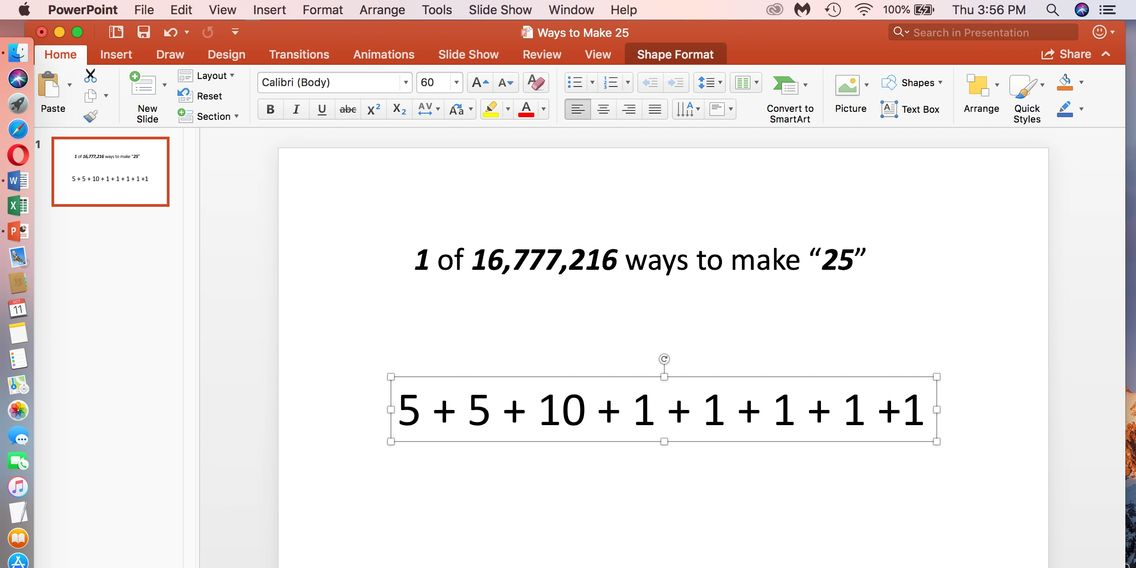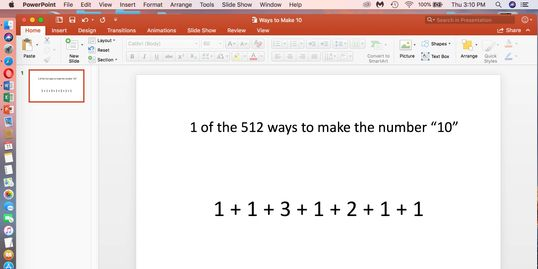Rumble and Tumble with Numbers: Ways to Make Numbers by Adding

# Ways to make a number by adding? Click on the link to OneDrive below.## Many Ways to Make a Number by Adding: Pre-algebra!#### Mathematical Thinking or Pre-Algebra

Did you know there are 16,777,216 ways to make the number "25" by adding only whole numbers, and using zero once?  Or, 2 to the power of (n-1) or 2 to the power of 24!#### 512 ways to make 10 by adding

It is not just math facts, but ways to think mathematically!  Yes, 1 + 1 + 3 + 1 + 2 + 1 + 1 =10.  Can you find some of the 512 ways to make the number "10" by adding whole numbers and using zero once?  This is pre-algebra at its best!#### Explore math beyond a few math facts like the 524,288 ways to make the number "20" by adding whole numbers, and using zero once!

How many things and ways do we count, add, subtract, multiply, and divide?  The computer only needs two fingers to count with since it uses base "2"  ( numbers 0, and 1  ).